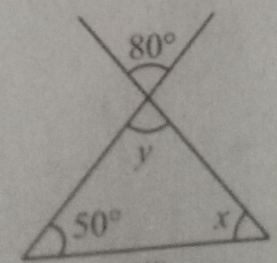"
">

# Find the value of the unknowns $x$ and $y" To find: The values of x and y in the given triangle Solution: Vertically Opposite Angles are the angles opposite each other when two lines cross.$ \angle y$and$80 ^{\circ} $are vertically opposite angles. So they are equal$ \angle y =80^{\circ}$Sum of angles in a triangle is$180^{\circ} $So$50^{\circ} + x + y = 180^{\circ}50^{\circ} + x + 80 = 180^{\circ} x  = 180^{\circ} - 50^{\circ} - 80^{\circ} x  = 50^{\circ}$So unknowns$ x  = 50^{\circ}$,$ y =80^{\circ}\$

Updated on: 10-Oct-2022

37 Views